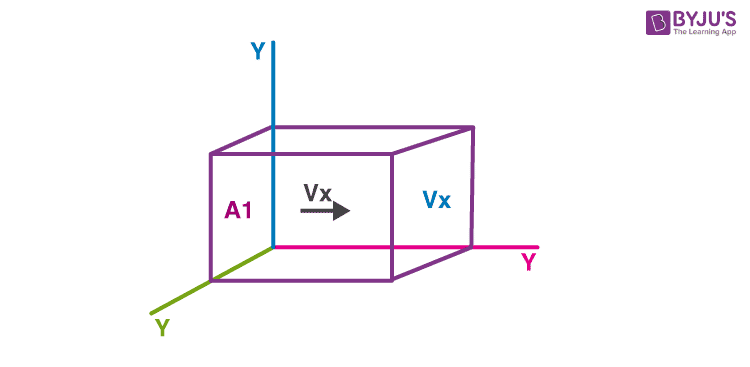# Calculating Pressure Of An Ideal Gas

Imagine an ideal gas contained in a cubical container. Let one corner of the cube be the origin O, and let the x, y, z-axes along the edges. Let A1and A2 be the parallel faces perpendicular to the x-axis. Consider a molecule moving with velocity v in the container. The components of velocity along the axes are vx, vy, and vz. Now, when the molecule is colliding with face A1, the x component of velocity is reversed while the y and z component of velocity remains unchanged (as per our assumption that the collisions are elastic).The change in momentum of the molecule is:
$$ΔP$$ = $$(-m v_x) – (m v_x)$$ = $$-2m v_x$$ ……….. (1)

Since the momentum remains conserved, the change in momentum of the wall is 2mvx

After the collision, the molecule travels towards the face $$A_2$$ with x component of the velocity equal to $$- v_x$$.

Distance travelled from $$A_1$$ to $$A_2$$ = $$L$$
Therefore, time = $$\frac{L}{v_x}$$

After collision with $$A_2$$, the molecule again travels to $$A_1$$. Thus, the time between two collisions is $$\frac{2L}{v_x}$$

Therefore, the number of collisions of the molecule per unit time:

$$n$$ = $$\frac{v_x}{2L}$$ ………………… (2)

Using equations 1 and 2,

The momentum imparted per unit time to the wall by the molecule:

$$ΔF$$ = $$nΔp$$

= $$\frac{m}{L} v_x^2$$

Therefore, total force on the wall A1 due to all the molecules is

$$F$$ = $$Ʃ \frac{m}{L} vx^2$$

= $$\frac{m}{L} Ʃ{v_x}^2$$

Now, $$Ʃ{v_x}^2$$ = $$Ʃ{v_y}^2$$ = $$Ʃ{v_z}^2$$ (symmetry)

= $$\frac{1}{3} Ʃv^2$$

Therefore, $$F$$ = $$\frac{1}{3} \frac{m}{L} Ʃv^2$$

Pressure is force per unit area so that

$$P$$ = $$\frac{F}{L^2}$$

= $$\frac{1}{3} \frac{M}{L^{3}} \frac{\sum v^{2}}{N}$$

= $$\frac{1}{3} \rho \frac{\sum v^{2}}{N}$$

Where,

$$M$$= total mass of gas

$$\rho$$ = density of the gas

Now, $$\frac{\sum v^{2}}{N}$$ is written as $$v^2$$ and is called the mean square speed.
$$P$$= $$\frac{1}{3}\rho v^{2}$$

To learn more on kinetic theory of gases, stay tuned with BYJU’S. Also, register to “BYJU’S – The Learning App” for loads of interactive, engaging Physics-related videos and an unlimited academic assist.